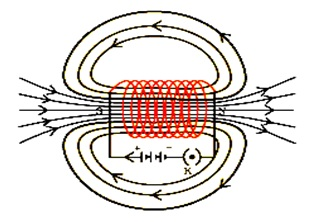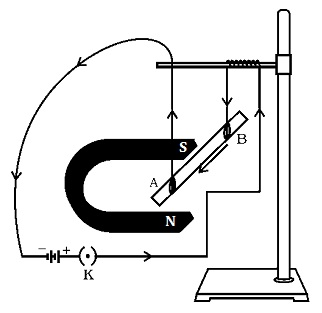⊜ Toppers Study
Study Materials:
CBSE NOTES ⇒ Class 10th ⇒ Science ⇒ 13. Magnetic Effects of Electric Current

## 13. Magnetic Effects of Electric Current

### Solenoid

Solenoid: A coil of many circular turns of insulated copper wire wrapped closely in the shape of a cylinder is called a solenoid.

Magnetic Field due to a Current in a Solenoid:

When electric current is passes through a solenoid, It's one end behaves as a magnetic north pole, while the other end behaves as the south pole.Properties of the field lines inside the solenoid:

• The field lines inside the solenoid are in the form of parallel straight lines.
• This indicates that the magnetic field is the same at all points inside the solenoid. Therefore, the field is uniform inside the solenoid.
• The field lines inside the solenoid are in the form of parallel straight lines. This property is used to make an electromagnet.
• A strong magnetic field produced inside a solenoid.

Electromagnet: A magnet is made by magnetic field produced inside a solenoid using magnetic materials like soft iron is called an electromagnet.

Some properties of electromagnet:

1. The magnetic field produced is generally very strong.
2. The strength of the magnetic-field can be controlled by controlling various factors such as the current and the number of turns in the solenoid.
3. The polarity of the magnet can be changed by reversing the direction of current while magnetic field is being produced by solenoid.

Differences between electromagnet and parmanent magnet:

 Electromagnet Permanent magnet 1. The magnetic field produced is generally very strong. 1. Generally the magnetic field produced is low and moderate. 2. The strength of the magnetic-field can be controlled by controlling various factors such as the current and the number of turns in the solenoid. 2. The strength of the magnetic-field of a permanent magnet is permanent but changes (decreases) with the temperature. 3. The polarity of the magnet can be changed by reversing the direction of current. 3. The polarity of the magnet can not be changed. 4. Normally soft iron is used for making electro magnets. 4. Cobalt, steel etc., are used for the purpose.

FORCE ON A CURRENT-CARRYING CONDUCTOR IN A MAGNETIC FIELD:

When a a strong horse-shoe magnet is placed in such a way that the rod lies between the two poles with the magnetic field directed upwards. For this the north pole of the magnet is kept vertically below and south pole is kept vertically above the aluminium rod. When a current is passed through the aluminium rod from end B to end A. It is observed that the rod is displaced. It is also observed when the direction of current flowing is reversed through rod the direction of displacement is also reversed.Conclusion:

(i) A magnetic field exerts a force on a magnet placed in the vicinity of the conductor.

(ii) A force is exerted on the current-carrying aluminium rod when it is placed
in a magnetic field.

(iii) The direction of force is also reversed when the direction of current through the conductor is reversed.

(iv) The direction of force acting on the current-carrying rod gets reversed when

the direction of current is reversed.

(v) The force on the conductor depends upon the direction of current and the direction of the magnetic field.

The force on the conductor:

The force on the conductor depends upon the flowing two things:

(i) The direction of current and

(ii) The direction of the magnetic field.

Other Pages of this Chapter:

### Select Your CBSE Classes

Important Study materials for classes 06, 07, 08,09,10, 11 and 12. Like CBSE Notes, Notes for Science, Notes for maths, Notes for Social Science, Notes for Accountancy, Notes for Economics, Notes for political Science, Noes for History, Notes For Bussiness Study, Physical Educations, Sample Papers, Test Papers, Mock Test Papers, Support Materials and Books.